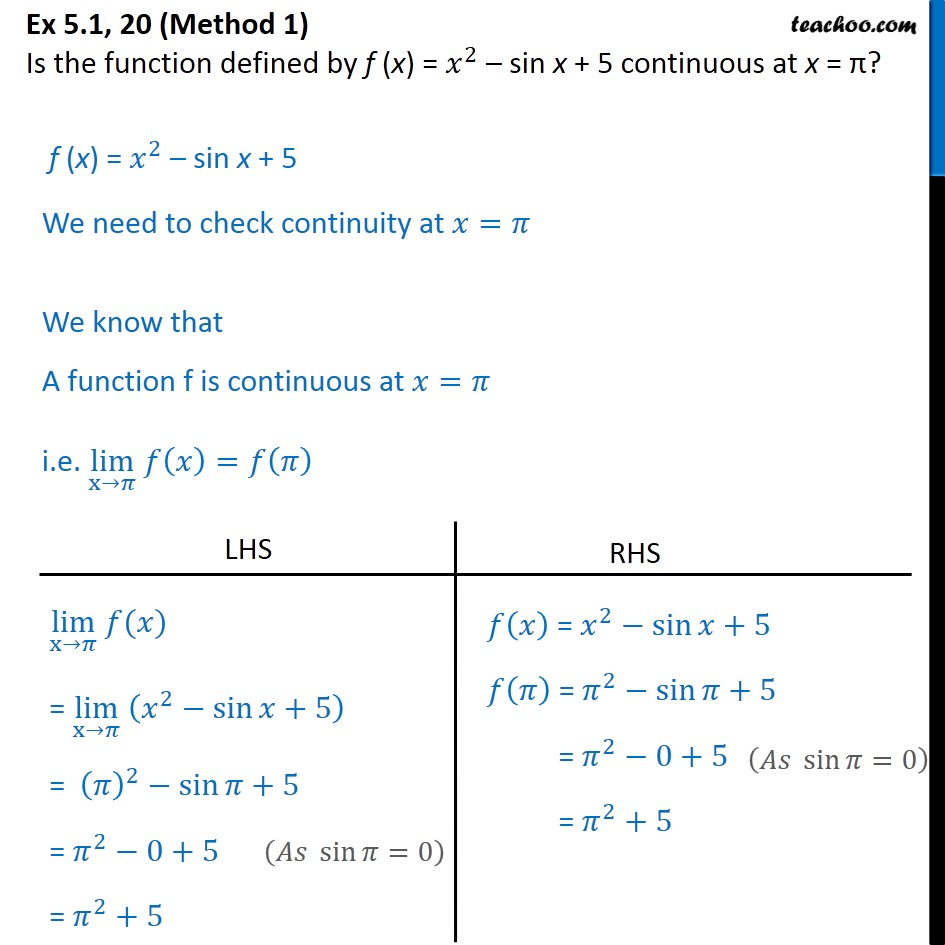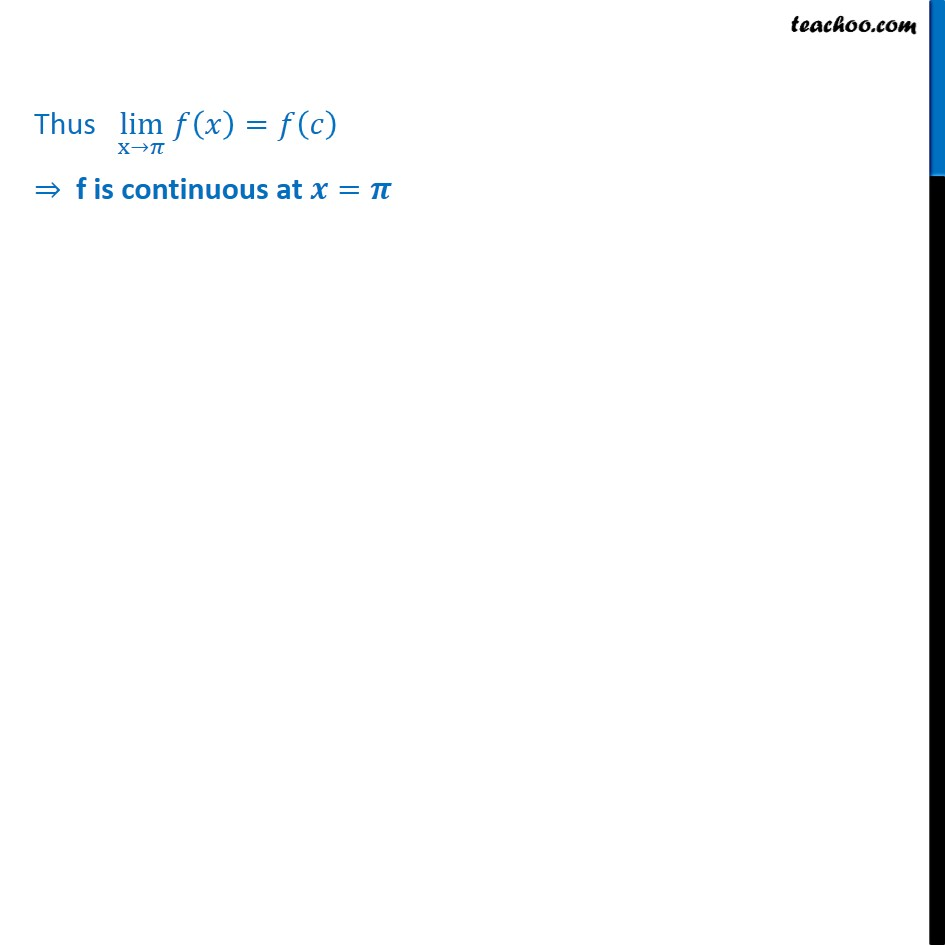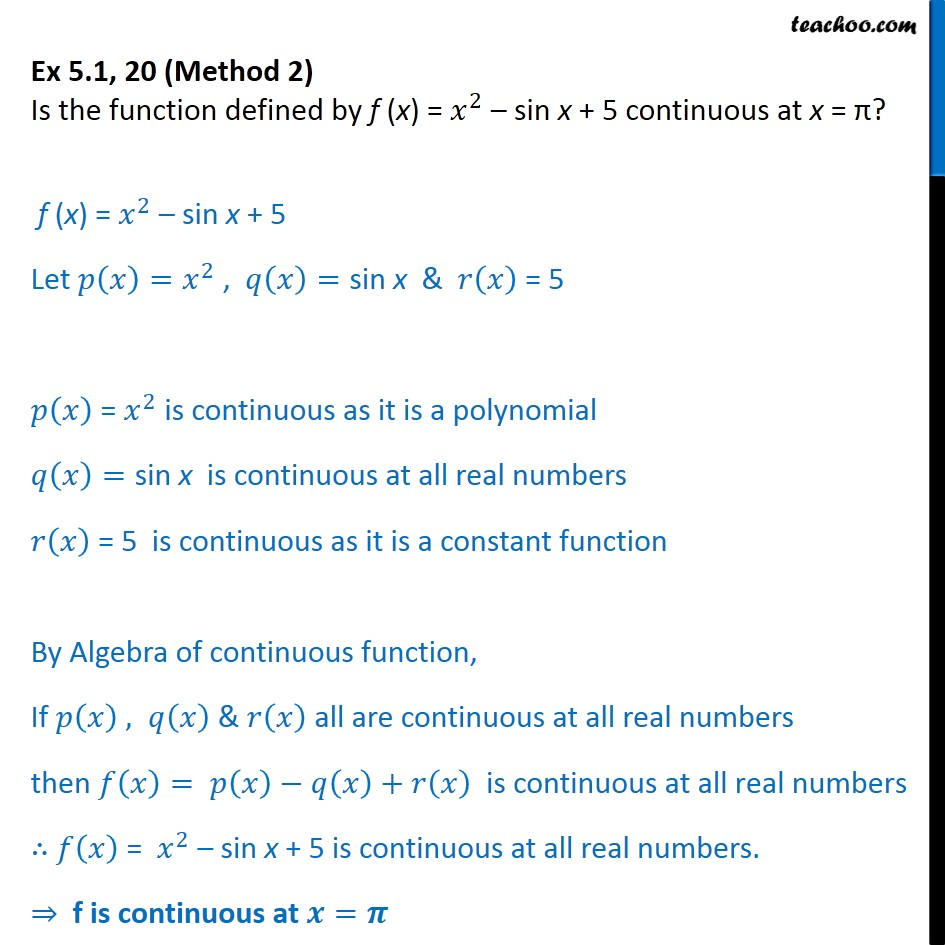1. Chapter 5 Class 12 Continuity and Differentiability
2. Serial order wise
3. Ex 5.1

Transcript

Ex 5.1, 20 (Method 1) Is the function defined by f (x) = 𝑥﷮2﷯ – sin x + 5 continuous at x = π? f (x) = 𝑥﷮2﷯ – sin x + 5 We need to check continuity at 𝑥=𝜋 We know that A function f is continuous at 𝑥=𝜋 i.e. lim﷮x→𝜋﷯ 𝑓 𝑥﷯=𝑓 𝜋﷯ Thus lim﷮x→𝜋﷯ 𝑓 𝑥﷯=𝑓 𝑐﷯ ⇒ f is continuous at 𝒙=𝝅 Ex 5.1, 20 (Method 2) Is the function defined by f (x) = 𝑥﷮2﷯ – sin x + 5 continuous at x = π? f (x) = 𝑥﷮2﷯ – sin x + 5 Let 𝑝 𝑥﷯= 𝑥﷮2﷯ , 𝑞 𝑥﷯=sin x & 𝑟 𝑥﷯ = 5 𝑝 𝑥﷯ = 𝑥﷮2﷯ is continuous as it is a polynomial 𝑞 𝑥﷯=sin x is continuous at all real numbers 𝑟 𝑥﷯ = 5 is continuous as it is a constant function By Algebra of continuous function, If 𝑝 𝑥﷯ , 𝑞 𝑥﷯ & 𝑟 𝑥﷯ all are continuous at all real numbers then 𝑓 𝑥﷯= 𝑝 𝑥﷯−𝑞 𝑥﷯+𝑟 𝑥﷯ is continuous at all real numbers ∴ 𝑓 𝑥﷯ = 𝑥﷮2﷯ – sin x + 5 is continuous at all real numbers. ⇒ f is continuous at 𝒙=𝝅

Ex 5.1

About the AuthorDavneet Singh
Davneet Singh is a graduate from Indian Institute of Technology, Kanpur. He has been teaching from the past 9 years. He provides courses for Maths and Science at Teachoo.